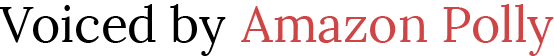# How to Teach Dividing Polynomials

 Would You Rather Listen to the Lesson?There are 3 different types of problems involving Dividing Polynomials. This lesson along with the lessons on Solving Systems of Equations are the two MOST difficult lessons for Algebra 1 students.

(Here are our FREE lessons on Solving Systems - Solving Systems by Graphing - Solving Systems by Substitution)

Here are the 3 Types of Dividing Polynomial Questions Your Students Will See

1: To divide monomials use the laws of exponents in division.

2: To divide a polynomial by a monomial, we use (a + b) / c = a/c + b/c

3: The last rule is to divide a polynomial by another polynomial with at least two terms. This type of division is applied only when the degree of the polynomial in the numerator is greater than or equal to the degree of polynomial in the denominator.

Do not try to do more than one of these in a day. Your students will get them confused with each other. These each have very specific step by step processes to solve. You do not want them taking bits and pieces from each and causing them the frustration to give up. Go slow! Long division is difficult for most students with numbers only now you are throwing variables and polynomials at them.

## Dividing Polynomials - PDFs

11-3 Guided Notes Teacher Edition (Members Only)

11-3 Lesson Plan (Members Only)

11-3 Online Activities (Members Only)

11-3 Video Lesson (Members Only)

## Simplifying Radicals - Word Docs & PowerPoints

To gain access to our editable content Join the Algebra 1 Teacher Community!
Here you will find hundreds of lessons, a community of teachers for support, and materials that are always up to date with the latest standards.